Download Presentation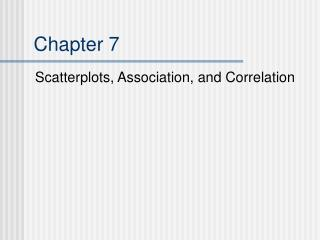Chapter 7

# Chapter 7 - PowerPoint PPT Presentation

Chapter 7. Scatterplots, Association, and Correlation. Examining Relationships. Relationship between two variables Examples: Height and Weight Alcohol and Body Temperature SAT Verbal Score and SAT Math Score High School GPA and College GPA. Two Types of Variables.I am the owner, or an agent authorized to act on behalf of the owner, of the copyrighted work described.
Download Presentation## Chapter 7

An Image/Link below is provided (as is) to download presentation

Download Policy: Content on the Website is provided to you AS IS for your information and personal use and may not be sold / licensed / shared on other websites without getting consent from its author.While downloading, if for some reason you are not able to download a presentation, the publisher may have deleted the file from their server.

- - - - - - - - - - - - - - - - - - - - - - - - - - E N D - - - - - - - - - - - - - - - - - - - - - - - - - -
Presentation TranscriptChapter 7

Scatterplots, Association, and Correlation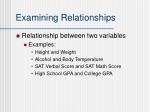Examining Relationships
• Relationship between two variables
• Examples:
• Height and Weight
• Alcohol and Body Temperature
• SAT Verbal Score and SAT Math Score
• High School GPA and College GPA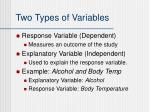Two Types of Variables
• Response Variable (Dependent)
• Measures an outcome of the study
• Explanatory Variable (Independent)
• Used to explain the response variable.
• Example: Alcohol and Body Temp
• Explanatory Variable: Alcohol
• Response Variable: Body Temperature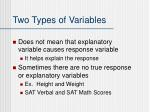Two Types of Variables
• Does not mean that explanatory variable causes response variable
• It helps explain the response
• Sometimes there are no true response or explanatory variables
• Ex. Height and Weight
• SAT Verbal and SAT Math Scores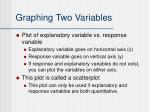Graphing Two Variables
• Plot of explanatory variable vs. response variable
• Explanatory variable goes on horizontal axis (x)
• Response variable goes on vertical axis (y)
• If response and explanatory variables do not exist, you can plot the variables on either axis.
• This plot is called a scatterplot
• This plot can only be used if explanatory and response variables are both quantitative.Scatterplots
• Scatterplots show patterns, trends, and relationships.
• When interpreting a scatterplot (i.e., describing the relationship between two variables) always look at the following:
• Overall Pattern
• Form
• Direction
• Strength
• Deviations from the Pattern
• Outliers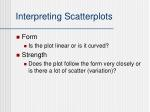Interpreting Scatterplots
• Form
• Is the plot linear or is it curved?
• Strength
• Does the plot follow the form very closely or is there a lot of scatter (variation)?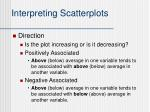Interpreting Scatterplots
• Direction
• Is the plot increasing or is it decreasing?
• Positively Associated
• Above (below) average in one variable tends to be associated with above (below) average in another variable.
• Negative Associated
• Above (below) average in one variable tends to be associated with below (above) average in another variable.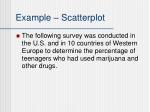Example – Scatterplot
• The following survey was conducted in the U.S. and in 10 countries of Western Europe to determine the percentage of teenagers who had used marijuana and other drugs.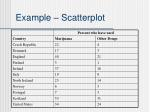Percent who have used

Country

Marijuana

Other Drugs

Czech Republic

22

4

Denmark

17

3

England

40

21

Finland

5

1

Ireland

37

16

Italy

19

8

North Ireland

23

14

Norway

6

3

Portugal

7

3

Scotland

53

31

United States

34

24

Example – ScatterplotExample – Scatterplot
• The variables are interchangeable in this example.
• In this example, Percent of Marijuana is being used as the explanatory variable (since it is on the x-axis).
• Percent of Other Drugs is being used as the response since it is on the y-axis.Example - Scatterplot
• The form is linear
• The strength is fairly strong
• The direction is positive since larger values on the x-axis yield larger values on the y-axisExample - Scatterplot
• Negative association
• Outside temperature and amount of natural gas usedCorrelation
• The strength of the linear relationship between two quantitative variables can be described numerically
• This numerical method is called correlation
• Correlation is denoted by rCorrelation
• A way to measure the strength of the linear relationship between two quantitative variables.Correlation
• Steps to calculate correlation:
• Calculate the mean of x and y
• Calculate the standard deviation for x and y
• Calculate
• Plug all numbers into formulaCalculating r.
• Femur (x) 38 56 59 63 74
• Humerus (y) 41 63 70 72 84
• Set up a table with columns for x, y, ,

, , , andx

y

38

41

-20

-25

400

625

500

56

63

-2

-3

4

9

6

59

70

1

4

1

16

4

63

72

5

6

25

36

30

74

84

16

18

256

324

288

290

330

0

0

686

1010

828

Calculating r.Calculating r
• Recall:
• So,Calculating r
• Recall:
• So,Calculating r.
• Put everything into the formula:Properties of r
• r has no units (i.e., just a number)
• Measures the strength of a LINEAR association between two quantitative variables
• If the data have a curvilinear relationship, the correlation may not be strong even if the data follow the curve very closely.Properties of r
• r always ranges in values from –1 to 1
• r = 1 indicates a straight increasing line
• r = -1 indicates a straight decreasing line
• r = 0 indicates no LINEAR relationship
• As r moves away from 0, the linear relationship between variables is strongerProperties of r
• Changing the scale of x or y will not change the value of r
• Not resistant to outliers
• Strong correlation ≠ Causation
• Strong linear relationship between two variables is NOT proof of a causal relationship!